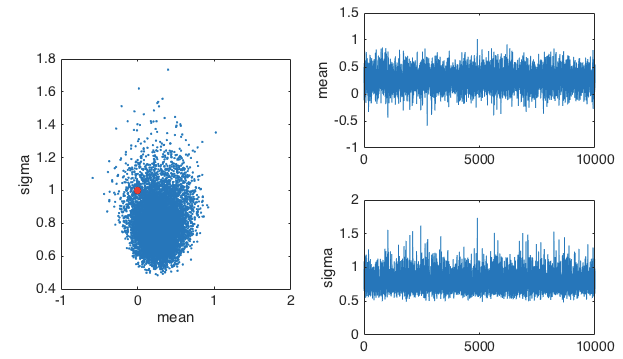So far we’ve had a look at rejection sampling and importance sampling. Here we take a quick look at slice sampling, although rather than implementing it ourselves, we will use the built in Matlab slicesample function.

Using our parameter estimation example, we will use slice sampling to estimate the mean and sigma of some samples from a normal distribution. Here is the code.

Which results in this plot of samples estimating the posterior P(params|data)